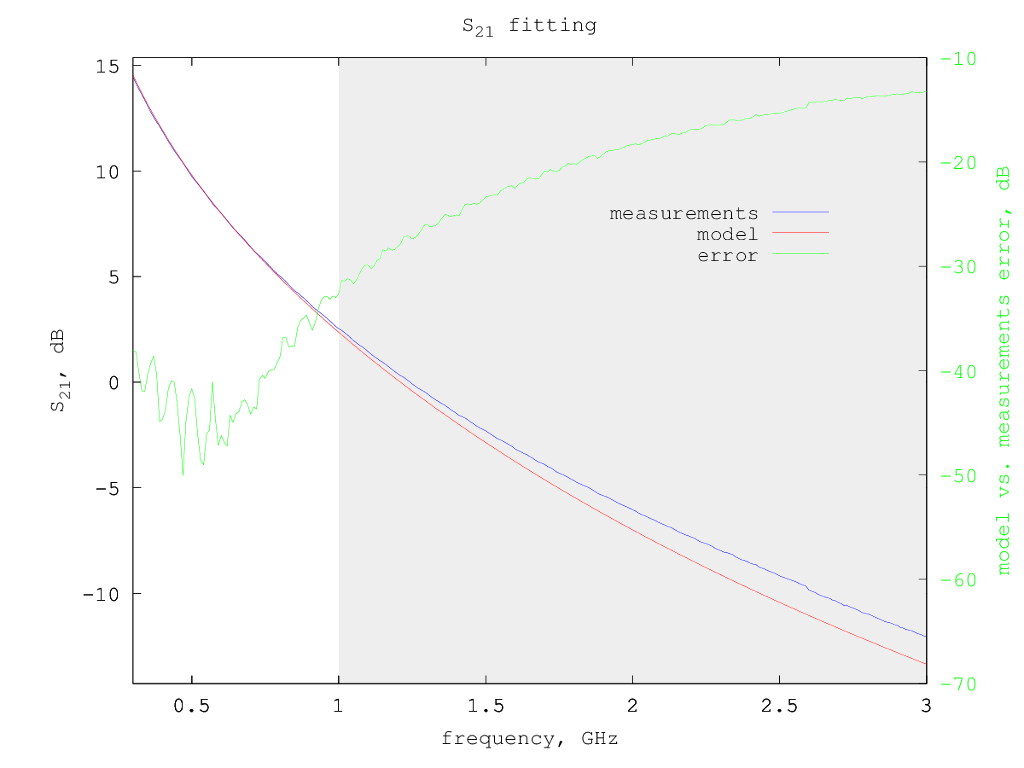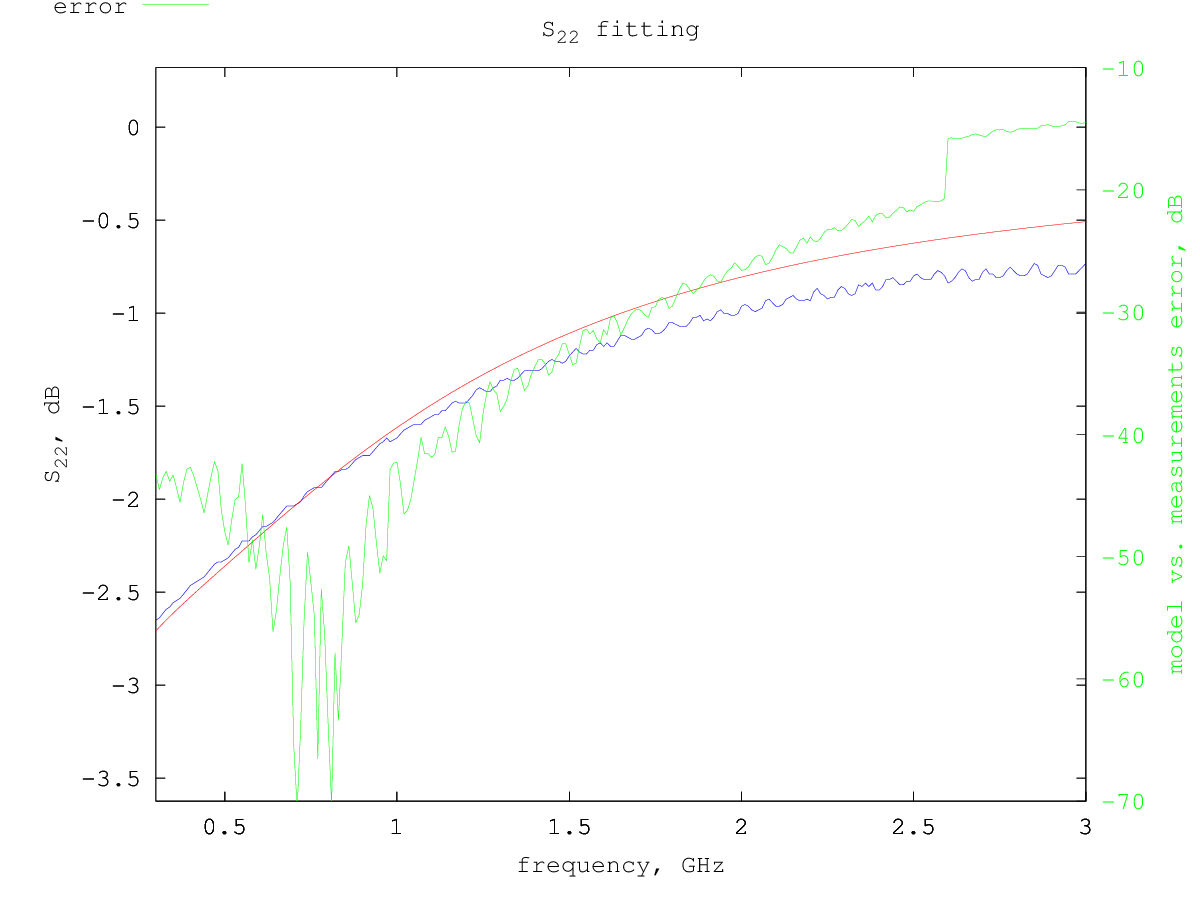# Renesas NE5500234 LDMOS model

Under construction...

The Renesas NE5500234 is a low power LDMOS.

# Model for Vdd=3.8 V and Idd= 100 mA

Typical S-parameters for this device can be found on the Renesas web site, for a supply voltage of 3.8 V and a drain current of 100 mA.

Using the extraction method previously described, considering only the S-parameters from 300 MHz to 1 GHz, the following small-signal model parameters are determined:

```Rg = 0.9 ohm
Rd = 0.53 ohm
Rs = 0.13 ohm
Lg = 0.57 nH
Ld = 0.61 nH
Ls = 0.012 nH
Cgs = 13.5 pF
Cds = 14.8 pF
Cgd = 2.3 pF
gm = 0.56 S
gds = 15.8 mS
```
So the equivalent small-signal circuit for this LDMOS at 100 mA drain current is the following:Smith chart with measured vs. model S-parameters:S-parameters fitting details - fitted region is in white background: